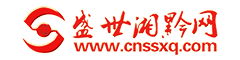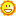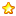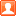湘黔新闻 湘黔要闻 城事热报 教育科技 湘黔网视 华商学院 名家访谈 品牌展播 娱乐时尚 娱乐快报 湘黔女郎 影视音乐 财富湘黔 财经新闻 产业园区 商会频道 湘黔文化 湖湘文化 金黔文化 湘黔公益 湘黔旅游 景区推荐 湘黔美食 城市生活
 搜索

# 一颗真心 书写忠诚 一片真情 融入田冲 图文/罗昭中

8820主题 3万积分30137发表于 2019-1-3 00:28:47 | 显示全部楼层 |阅读模式[size=0.34]驻村八个月，240个昼夜，吃住在村，走访进村，交流入户，见面到人。全村350户，1070人，除外出务工人员外，在家村民保证家家走到，户户进屋，人人见面，个个认识。烈日炎炎戴草帽，天雨路滑穿水靴；快递站点来回跑，背篼风采穿乡村；手把锄头来挥舞，脚步穿行访民情；尘土飞溅晓民意，扎根山村铸忠诚。这是检查改建好的进村主道，经过两个月的努力，拓宽了原来的湾急坡陡死结，为村民消除了安全隐患，我们的笑容也是发自内心。[size=0.34]带领村干部看望生病的85岁老人何均珍[size=0.34]带领村干部看望马路槽摔伤骨折的77岁老人[size=0.34]与村干部一起检查硬化好的进村主道[size=0.34]带领单位医生为摔伤老人入户义诊[size=0.34]带领医院同事入户为78岁老人义诊[size=0.34]陪同单位领导看望78岁老人[size=0.34]带领单位同事慰问幼儿园小朋友，送去玩具、爱心牛奶[size=0.34]协调康复医院同事为入托儿童每日提供一盒爱心牛奶[size=0.34]污水横流中，保护孩子不受泥泞[size=0.34]秋风凌厉中为耄耋老人送去暖被，传递党的温暖[size=0.34]看望富有正能量，有人在阻工，他却烧水送工地，坚持每日清扫路面的78岁老人黄尚武[size=0.34]孩子们，爱心牛奶到了[size=0.34]为村民接送孩子[size=0.34]为孩子们采购爱心牛奶[size=0.34]看望耄耋老人[size=0.34]利用火炉会宣讲党的好政策[size=0.34]小朋友们后面的区域柜等物件由驻村干部募捐，这不，你看我们的孩子们在驻村帮扶幼儿园入托好开心[size=0.34]检查施工现场，协调纠纷[size=0.34]为耄耋老人义诊[size=0.34]与贵阳幼儿师范学院领导座谈帮扶田冲幼儿园事宜[size=0.34]小朋友穿上书记伯伯赠送的棉鞋好开心[size=0.34]坚守在施工现场，督查质量[size=0.34]孩儿们，你们倒是放开我啊[size=0.34]赠送本人警用大衣给退役军人[size=0.34]自费为帮扶田冲的友人赠送锦旗[size=0.34]纯真小朋友与我一家亲[size=0.34]为老人解答疑难[size=0.34]勘验公立山村幼儿园建设方案[size=0.34]检查道路施工进度[size=0.34]到供电所为村民协调改线方案[size=0.34]会商道路施工方案[size=0.34]接受上级督查[size=0.34]为83岁老人送去寒衣[size=0.34]远赴桐梓为孩子们募集物资[size=0.34]利用周末行走各地为田冲募捐各种助学物资[size=0.34]检查危房[size=0.34]与县教育局会商幼儿园改建方案[size=0.34]走访贫困户[size=0.34]硬化后漂亮的道路[size=0.34]看望老人[size=0.34]自费捐赠音响给幼儿园小朋友[size=0.34]有了书记伯伯捐赠的音响，以后孩子们可以大声唱歌了[size=0.34]对接社会爱心人士到田冲帮扶[size=0.34]扶老携幼乃做人本份[size=0.34]关爱帮扶留守儿童[size=0.34]冒雨检查险情[size=0.34]与受诊老年朋友合影[size=0.34]利用义诊现场宣讲党的政策[size=0.34]看望品学兼优的留守儿童[size=0.34]快递哥一枚[size=0.34]搬运工一个[size=0.34]国庆节期间坚守田冲协调施工[size=0.34]现场查勘维修方案[size=0.34]关注留守儿童[size=0.34]搀扶耄耋老人[size=0.34]清理环境卫生[size=0.34]锦旗一方表谢意[size=0.34]贵大美女教授捐助蹦床一台[size=0.34]遵义汇聚爱心助学协会到田冲慰问帮扶[size=0.34]现场查勘施工方案[size=0.34]坚守施工现场[size=0.34]协调处理施工纠纷[size=0.34]现场协调处理纠纷[size=0.34]小朋友看见驻村干部宛如明星[size=0.34]为幼儿园募捐购买的区域柜[size=0.34]锦旗一方显真情[size=0.34]亲自搬运为幼儿募集的过冬棉鞋[size=0.34]冒雨检查山塘水库[size=0.34]坚持清理环境卫生[size=0.34]向省局领导汇报驻村工作[size=0.34]走访查证，体恤民情[size=0.34]背篼哥一枚[size=0.34]草帽哥一个[size=0.34]赠送寒衣为老人亲自穿上[size=0.34]搀扶老人进行体检[size=0.34]主道改造也具雏形[size=0.34]仔细检查垮塌路段，安排对接维修方案[size=0.34]主道硬化已经完成，田冲即将迎来新时代[size=0.34]为耄耋老人送去冬被，传递党的温暖
《盛世湘黔网》www.cnssxq.com传承湖湘文化  网聚湘黔精英，加强民间交流，助推湘黔发展

 本版积分规则 回帖后跳转到最后一页